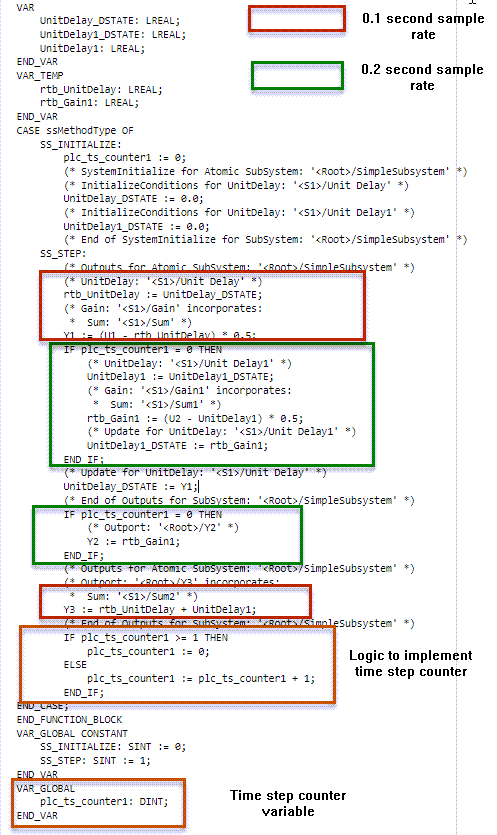# Generate Structured Text Code for a Simulink Subsystem that Has Multirate Components

This example shows how to model, simulate, and generate code for a Simulink® subsystem that has components running at different sample times (multirate). Multirate systems are common in industrial applications and programable logic controllers(PLCs). For example, within a PLC there is a task to collect information from sensors to control a process that runs at a slower rate and the actual logic to control the process that runs at a faster rate. To setup your multirate model for simulation and code generation, see Generating Simulink PLC Coder Structured Text Code for Multirate Models.

### Model Description

The model has a simple subsystem with components running at different sample rates. The input sine wave to `U1` runs at a 0.1 second sample rate and the input sine wave to `U2` runs at a 0.2 second sample rate. Output `Y1` is calculated using `U1`, output `Y2` is calculated using `U2`, and output `Y3` is calculated using both `U1` and `U2`. This image shows the components of the multirate subsystem. The blocks in red run at a 0.1 second sample rate and the blocks in green run at a 0.2 second sample rate. When using unit delay blocks in multirate models set the unit delay block `Sample time` parameter to `-1`.Open the model:

```load_system('plcdemo_multirate'); open_system('plcdemo_multirate/SimpleSubsystem');```

### Generate Code

To generate structured text code, do one of the following:

• Open the PLC Coder app. Select the `SimpleSubsystem` block and click `Generate PLC Code`.

• Use the `plcgeneratecode` function:

`plcgeneratecode('plcdemo_multirate/SimpleSubsystem');`
```### Generating PLC code for 'plcdemo_multirate/SimpleSubsystem'. ### Using model settings from 'plcdemo_multirate' for PLC code generation parameters. ### Begin code generation for IDE codesys23. ### Emit PLC code to file. ### Creating PLC code generation report plcdemo_multirate_codegen_rpt.html. ### PLC code generation successful for 'plcdemo_multirate/SimpleSubsystem'. ### Generated files: plcsrc/plcdemo_multirate.exp ```

### Structure of Generated Code

The generated structured text code for the multirate subsystem consists of these components:

• Time step counter variable: This portion of the code contains variable declarations for the time step counter that implements the different sample times. For subsystems that have `n` different sample times, the generated code has `n-1` time step counter variables that correspond to the `n-1` slower sample rates.

• Logic to implement time step counter: This portion of the code contains the code that implements the counter to implement the different sample times.

• Code blocks running at different sample rates: These are portions of the code that run at different sample rates. For example, in this image the blocks of code in green run at a 0.2 second sample rate and the blocks of code in red run at a 0.1 second sample rate.

This image shows the different components of the generated structured text code.When you deploy the generated code, you must run the code at the fastest sample rate. For example, when deploying the generated code for this model run the code at a 0.1 second sample rate.